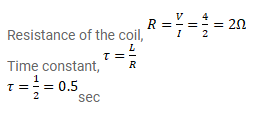# An inductor-coil carries a steady-current of

Question:

An inductor-coil carries a steady-current of $2.0 \mathrm{~A}$ when connected across an ideal battery of emf $4.0 \mathrm{~V}$. It its inductance is $1.0$ $\mathrm{H}$, find the time constant of the circuit.

Solution: